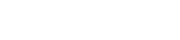homecontact us# Frequently Asked Questions - Web Ranking ™

 Q: What is and How is the Ranking Score calculated A: The ranking score is calculated by the following formula: ( count ^ 1/3 * eng_weight * pos_weight ) / 10,000 It is an indicator which measures how well a particular web site's ranking. This is calculated after analyzing the position on the search engine by using the keyword/phrase. count = Number of sites found by the given keyword by the search engine eng_weight = Engine weight, weight of a search engine you have set in the "Score" tab pos_weight = Position weight, weight of a position you have set in the "Score" tab

# Ranking Score FAQWhat is and How is the Ranking Score calculated?What is KEI? MORE FAQs